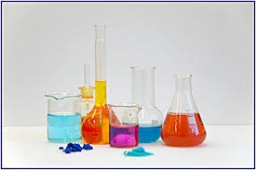# Dilute 8392

I have 500ml of 31% acid and must dilute it to 5%.
How many ml of water do I need to add?

x =  2600 ml

### Step-by-step explanation:

500·0.31 = (500+x) · 0.05

0.05x = 130

x = 130/0.05 = 2600

x = 2600

Our simple equation calculator calculates it.Did you find an error or inaccuracy? Feel free to write us. Thank you!

Tips for related online calculators